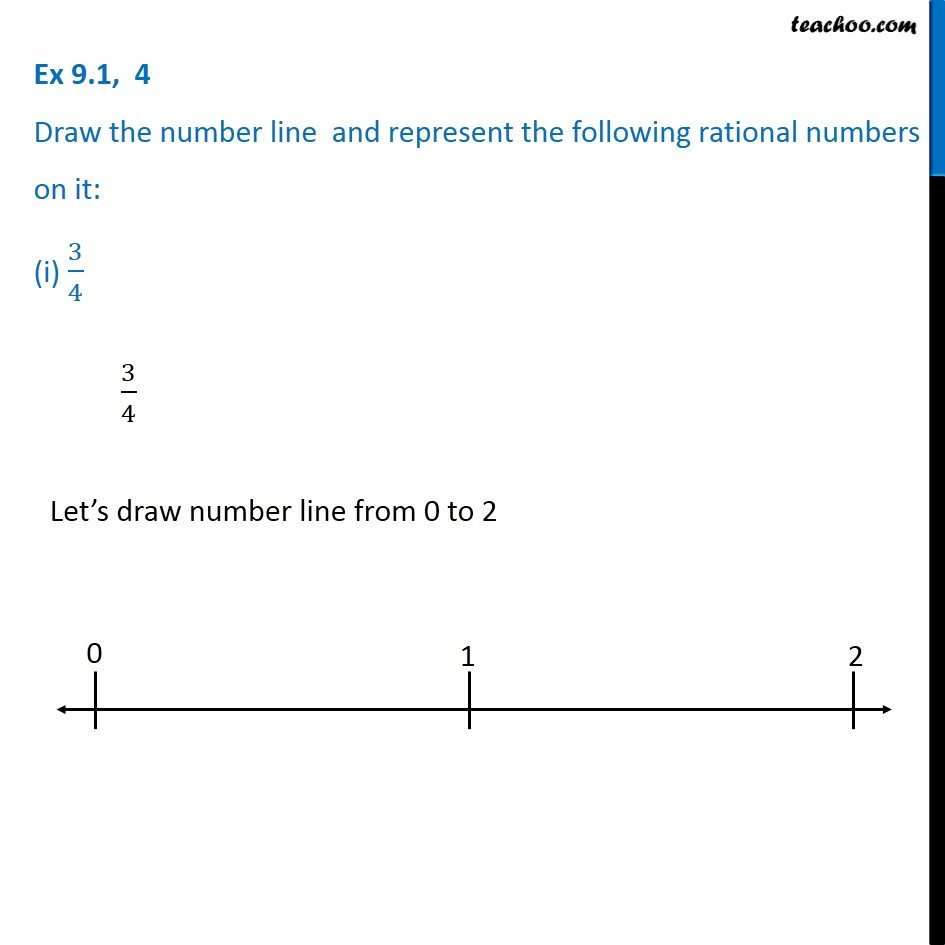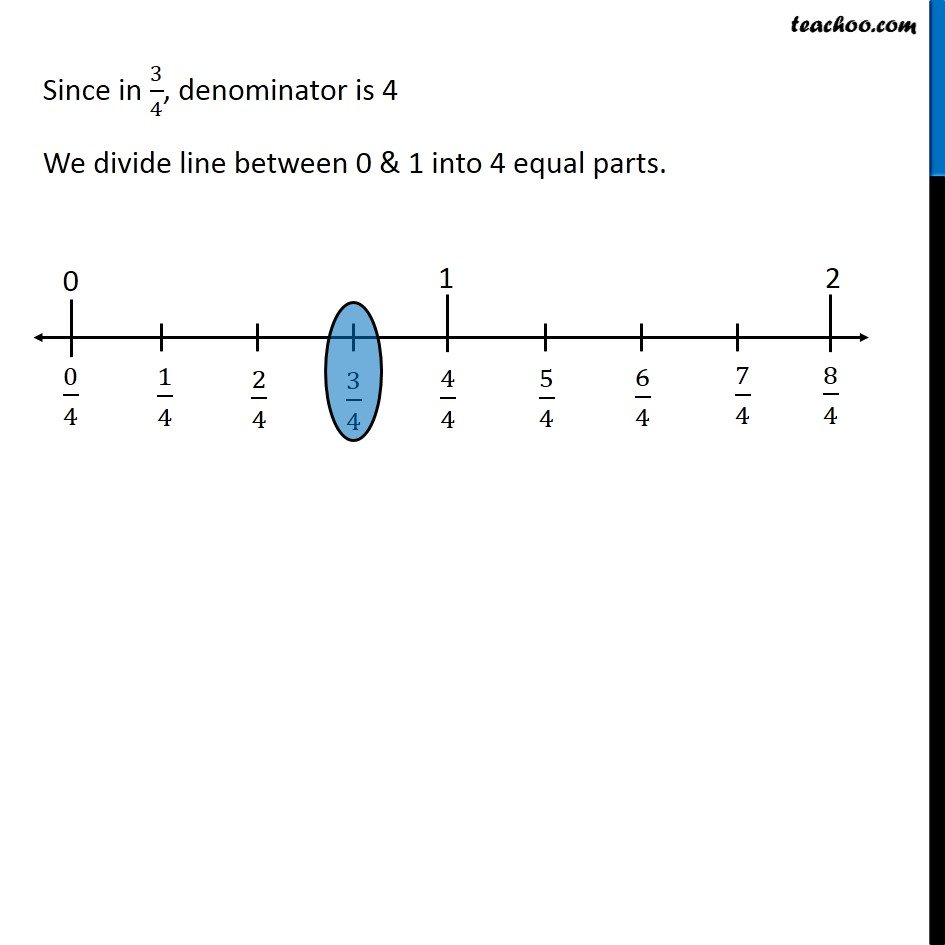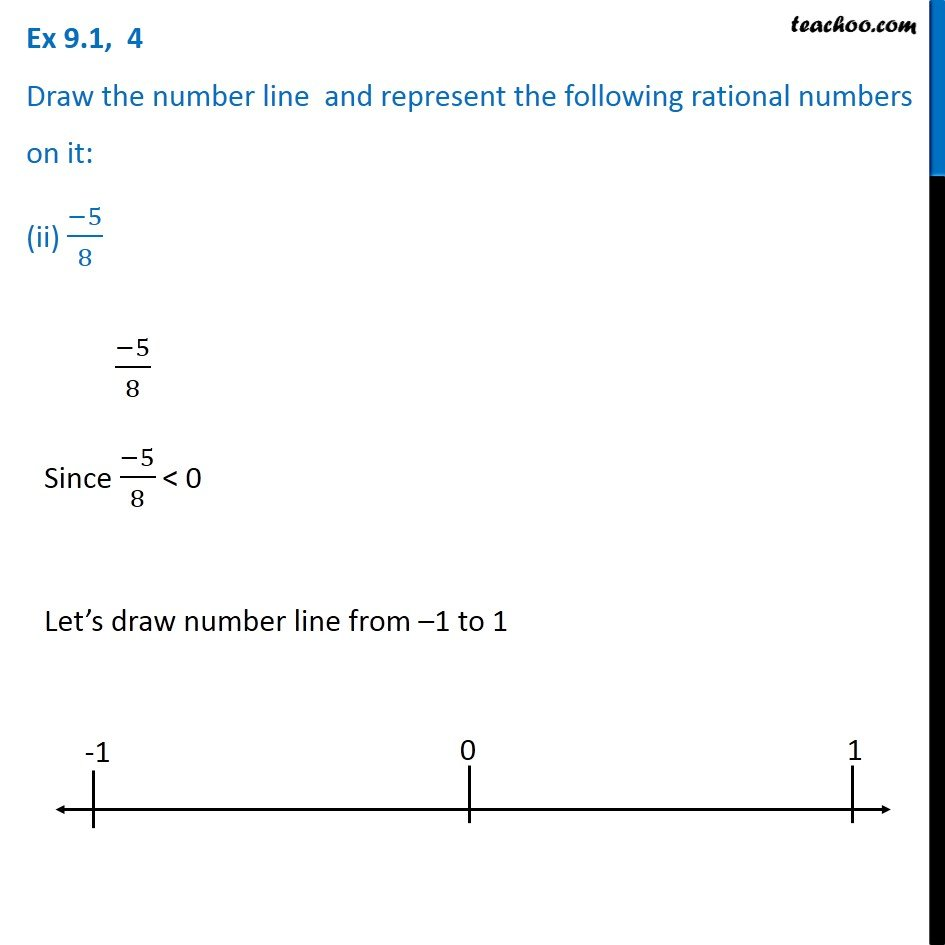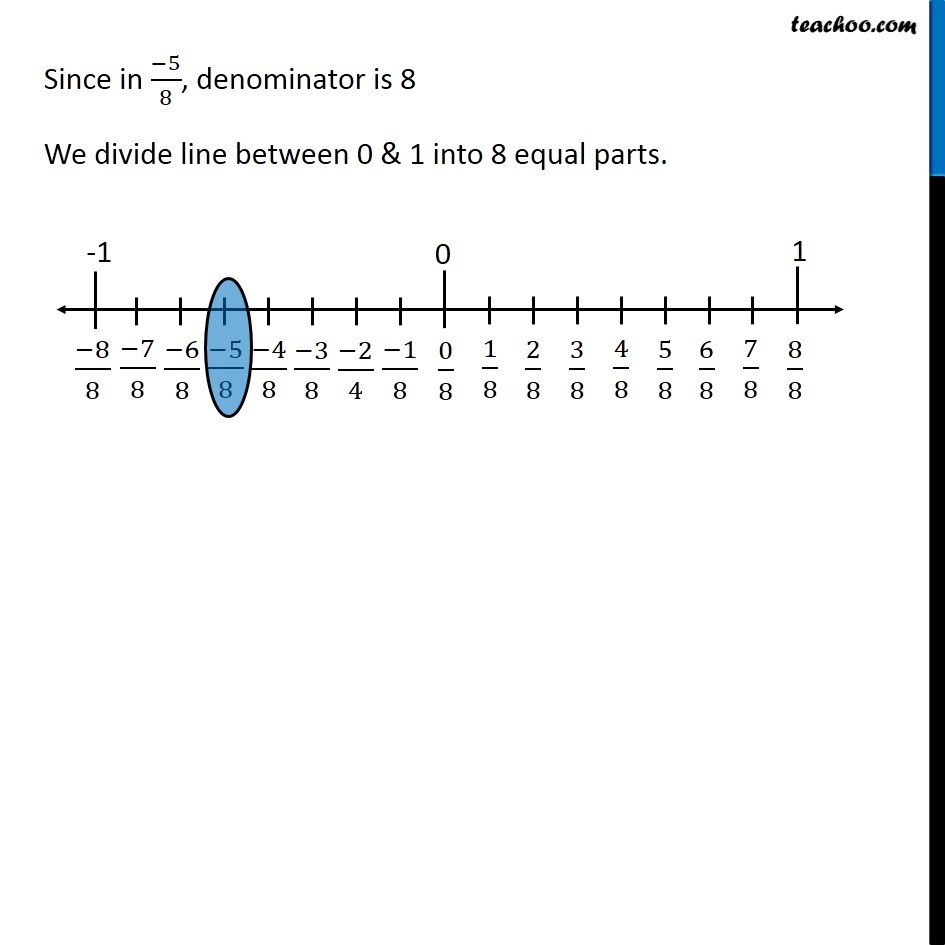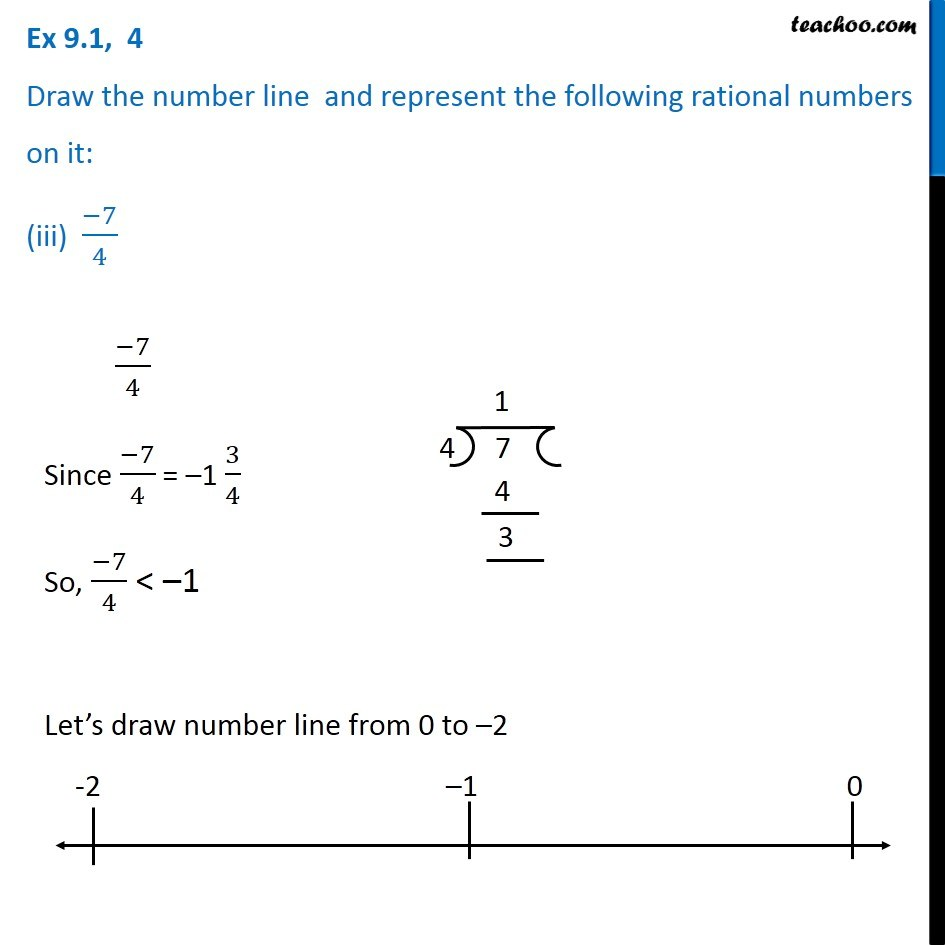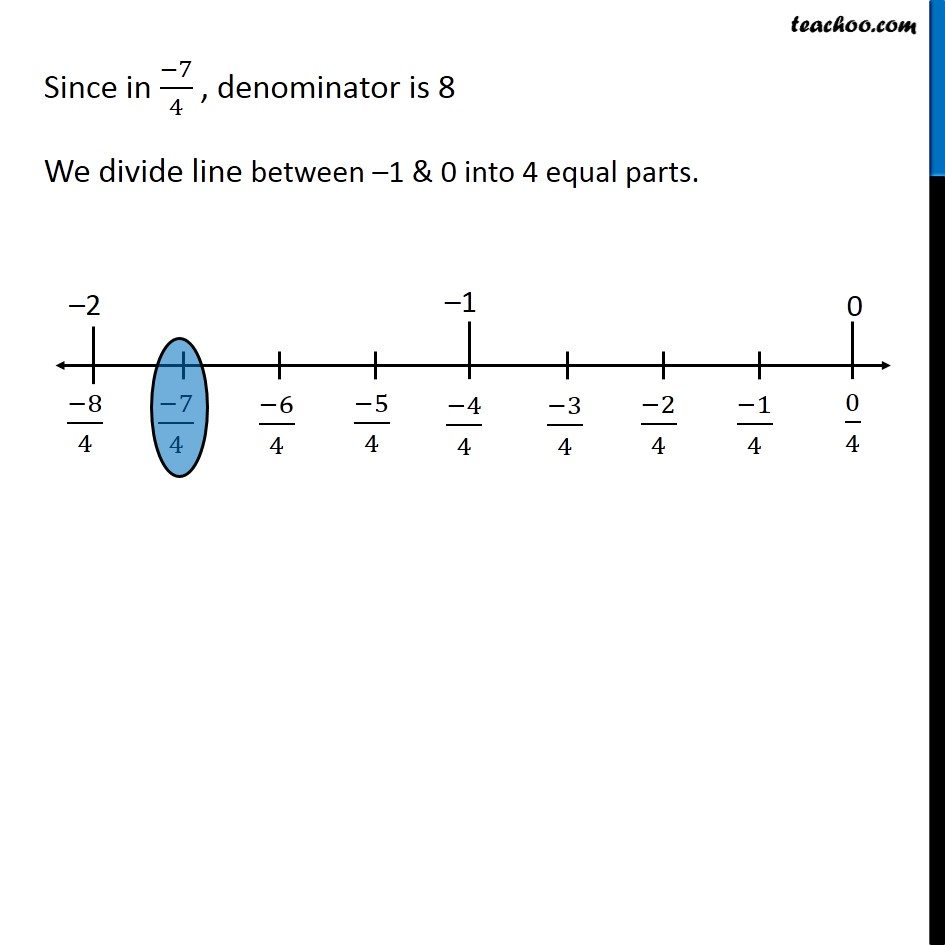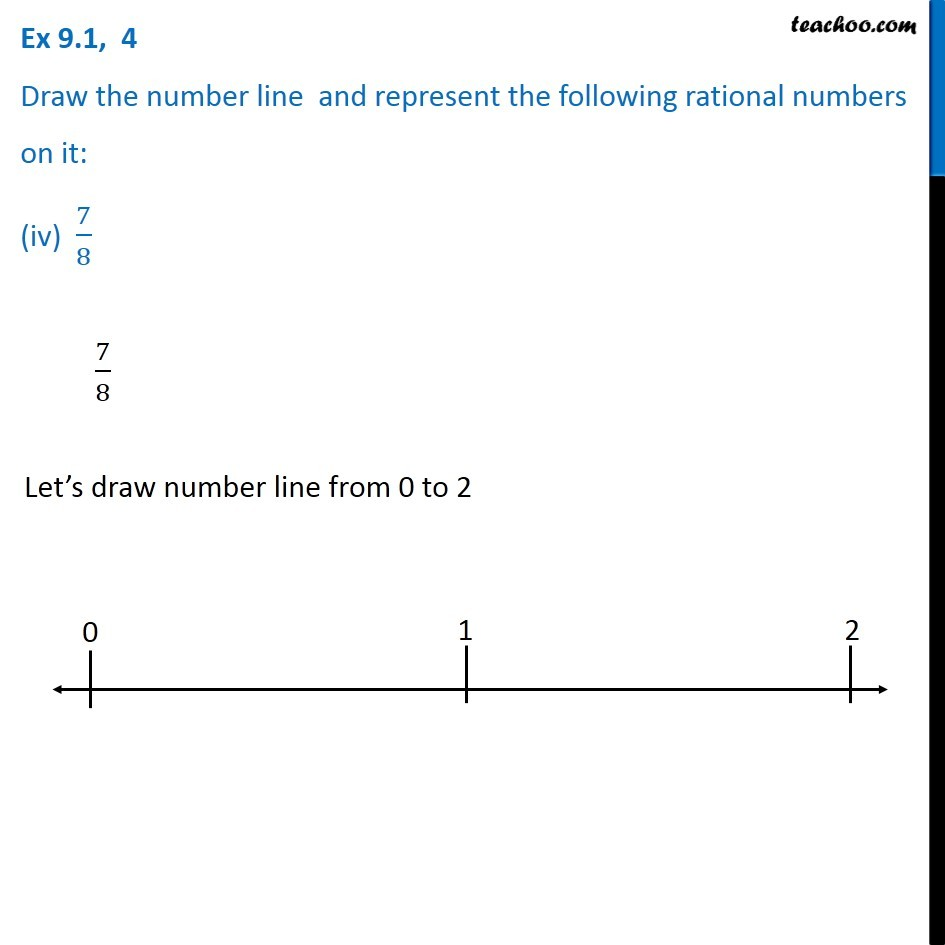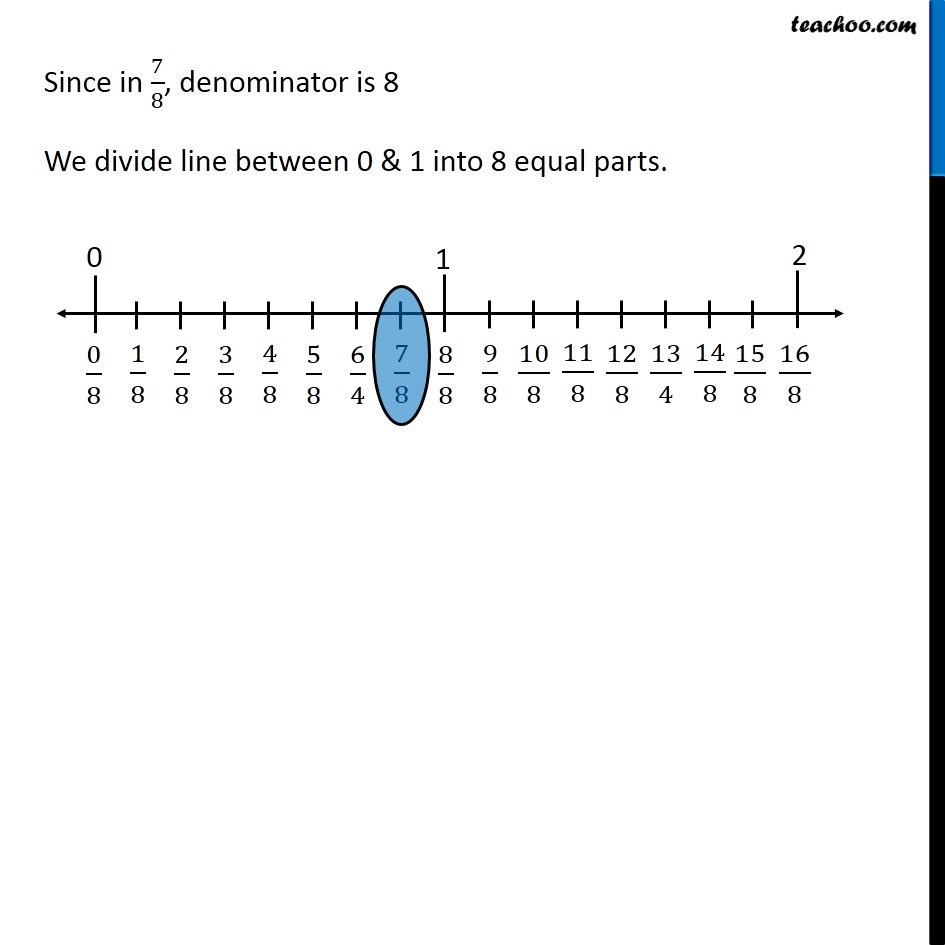1. Chapter 9 Class 7 Rational Numbers
2. Serial order wise
3. Ex 9.1

Transcript

Ex 9.1, 4 Draw the number line and represent the following rational numbers on it: (i) 3/4 3/4 Let’s draw number line from 0 to 2 Since in 3/4, denominator is 4 We divide line between 0 & 1 into 4 equal parts. Ex 9.1, 4 Draw the number line and represent the following rational numbers on it: (ii) (−5)/8 (−5)/8 Since (−5)/8 < 0 Let’s draw number line from –1 to 1 Since in (−5)/8, denominator is 8 We divide line between 0 & 1 into 8 equal parts. Ex 9.1, 4 Draw the number line and represent the following rational numbers on it: (iii) (−7)/4 (−7)/4 Since (−7)/4 = –1 3/4 So, (−7)/4 < –1 Let’s draw number line from 0 to –2 Since in (−7)/4 , denominator is 8 We divide line between –1 & 0 into 4 equal parts. Ex 9.1, 4 Draw the number line and represent the following rational numbers on it: (iv) 7/8 7/8 Let’s draw number line from 0 to 2 Since in 7/8, denominator is 8 We divide line between 0 & 1 into 8 equal parts.

Ex 9.1

About the AuthorDavneet Singh
Davneet Singh is a graduate from Indian Institute of Technology, Kanpur. He has been teaching from the past 10 years. He provides courses for Maths and Science at Teachoo.